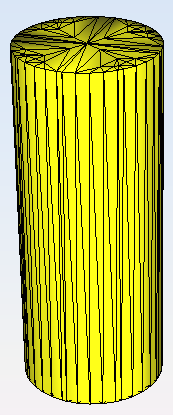# Create Mesh

In the PO module, the mesh generation is a required step that is performed immediately before simulating. In the Meshing Parameters window, represented in the next Figure, the following options are available:
In the Meshing Options panel the following options are available:
• Number of processors: To set the number of processors for the meshing process.
• Meshing Strategy
• Regular Meshing: The size of the generated elements is selected by the user. Regular meshing is the common algorithm used in other modules such as MOM. It provides a mesh of triangles of similar sizes with a distribution as uniform as possible. It enables the user to set the exact size edge of the triangles to be generated, given in lambda units. However, a huge number of mesh elements may results when the geometry contains large surfaces.
• Multilevel: Use Multilevel to generate the mesh automatically in several sequenced steps. This option is usually more efficient (in terms of runtime) than the common mesh generation option, so it is selected by default. However, there may be minor differences between the meshes obtained with and without the Multilevel mode.
• Edge size: The desired size for the edge of the elements, in wavelengths.
• Curvature Meshing:

Using curvature meshing we get the meshed geometry defined in terms of triangles. This geometry should be very similar to the original geometry. We can force that the meshed geometry be close to the original one using the surface error (in wavelengths). In the mesher we have default values for this magnitude but the user can change it. The mesher tries to minimize the number of triangles of a meshed geometry such that the distance from any point of this geometry to the original one is smaller than the “selected surface error”.

If we have a cube, say the original geometry is defined by six flat quadrangles the meshed geometry is composed by twelve triangles. In this case the distance of any point of the meshed geometry to the original one is null, say both geometries are identical for every frequency.

If an sphere of 1 m of radius at 300 MHz the “selected value” using the defaults values is 0.08*wavelength = 8.0 cm. The mesh is composed by 104 triangles. If you increase the frequency we obtain the same mesh until about 0.7 GHz. Due to topological restriction the number of elements of a mesh of triangle can not increases continuously, say we can not go from 104 triangles to 105 triangles for the sphere (next for the sphere can be 174 triangles).

The Distance error error is other parameter of the meshing by curvature. It only applies to flat facets. The longer edge of the planar surface is split by this parameter. For instance if you set 10 for the cube case you get 12*(102) = 1200 triangles. By default we have 0.01, that is, if this parameter is lower than 1.0 not division of flat facets is consider.

The table below shows a comparison of meshing criteria with a different configuration for meshing a cylinder with a radius of 0.25 m. and height of 1 m. at 3 GHz.
 Regular 1 lambda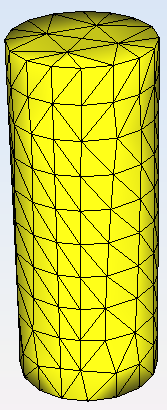0.5 lambda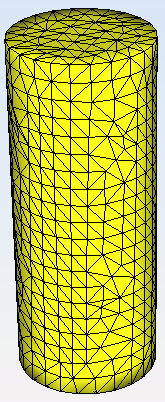0.01 lambda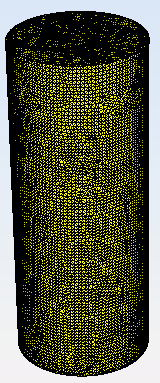Curvature 0.2 surface error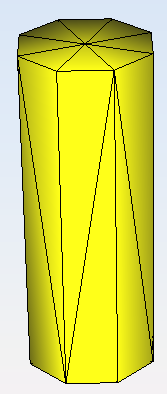0.1 surface error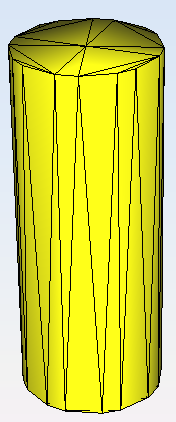0.01 surface error